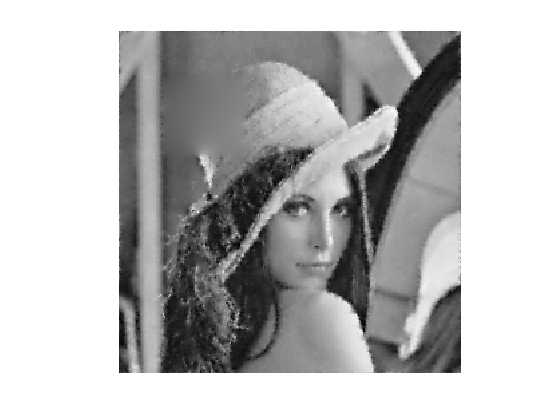$\newcommand{\NN}{\mathbb{N}} \newcommand{\CC}{\mathbb{C}} \newcommand{\GG}{\mathbb{G}} \newcommand{\LL}{\mathbb{L}} \newcommand{\PP}{\mathbb{P}} \newcommand{\QQ}{\mathbb{Q}} \newcommand{\RR}{\mathbb{R}} \newcommand{\VV}{\mathbb{V}} \newcommand{\ZZ}{\mathbb{Z}} \newcommand{\FF}{\mathbb{F}} \newcommand{\KK}{\mathbb{K}} \newcommand{\UU}{\mathbb{U}} \newcommand{\EE}{\mathbb{E}} \newcommand{\Aa}{\mathcal{A}} \newcommand{\Bb}{\mathcal{B}} \newcommand{\Cc}{\mathcal{C}} \newcommand{\Dd}{\mathcal{D}} \newcommand{\Ee}{\mathcal{E}} \newcommand{\Ff}{\mathcal{F}} \newcommand{\Gg}{\mathcal{G}} \newcommand{\Hh}{\mathcal{H}} \newcommand{\Ii}{\mathcal{I}} \newcommand{\Jj}{\mathcal{J}} \newcommand{\Kk}{\mathcal{K}} \newcommand{\Ll}{\mathcal{L}} \newcommand{\Mm}{\mathcal{M}} \newcommand{\Nn}{\mathcal{N}} \newcommand{\Oo}{\mathcal{O}} \newcommand{\Pp}{\mathcal{P}} \newcommand{\Qq}{\mathcal{Q}} \newcommand{\Rr}{\mathcal{R}} \newcommand{\Ss}{\mathcal{S}} \newcommand{\Tt}{\mathcal{T}} \newcommand{\Uu}{\mathcal{U}} \newcommand{\Vv}{\mathcal{V}} \newcommand{\Ww}{\mathcal{W}} \newcommand{\Xx}{\mathcal{X}} \newcommand{\Yy}{\mathcal{Y}} \newcommand{\Zz}{\mathcal{Z}} \newcommand{\al}{\alpha} \newcommand{\la}{\lambda} \newcommand{\ga}{\gamma} \newcommand{\Ga}{\Gamma} \newcommand{\La}{\Lambda} \newcommand{\Si}{\Sigma} \newcommand{\si}{\sigma} \newcommand{\be}{\beta} \newcommand{\de}{\delta} \newcommand{\De}{\Delta} \renewcommand{\phi}{\varphi} \renewcommand{\th}{\theta} \newcommand{\om}{\omega} \newcommand{\Om}{\Omega} \renewcommand{\epsilon}{\varepsilon} \newcommand{\Calpha}{\mathrm{C}^\al} \newcommand{\Cbeta}{\mathrm{C}^\be} \newcommand{\Cal}{\text{C}^\al} \newcommand{\Cdeux}{\text{C}^{2}} \newcommand{\Cun}{\text{C}^{1}} \newcommand{\Calt}{\text{C}^{#1}} \newcommand{\lun}{\ell^1} \newcommand{\ldeux}{\ell^2} \newcommand{\linf}{\ell^\infty} \newcommand{\ldeuxj}{{\ldeux_j}} \newcommand{\Lun}{\text{\upshape L}^1} \newcommand{\Ldeux}{\text{\upshape L}^2} \newcommand{\Lp}{\text{\upshape L}^p} \newcommand{\Lq}{\text{\upshape L}^q} \newcommand{\Linf}{\text{\upshape L}^\infty} \newcommand{\lzero}{\ell^0} \newcommand{\lp}{\ell^p} \renewcommand{\d}{\ins{d}} \newcommand{\Grad}{\text{Grad}} \newcommand{\grad}{\text{grad}} \renewcommand{\div}{\text{div}} \newcommand{\diag}{\text{diag}} \newcommand{\pd}{ \frac{ \partial #1}{\partial #2} } \newcommand{\pdd}{ \frac{ \partial^2 #1}{\partial #2^2} } \newcommand{\dotp}{\langle #1,\,#2\rangle} \newcommand{\norm}{|\!| #1 |\!|} \newcommand{\normi}{\norm{#1}_{\infty}} \newcommand{\normu}{\norm{#1}_{1}} \newcommand{\normz}{\norm{#1}_{0}} \newcommand{\abs}{\vert #1 \vert} \newcommand{\argmin}{\text{argmin}} \newcommand{\argmax}{\text{argmax}} \newcommand{\uargmin}{\underset{#1}{\argmin}\;} \newcommand{\uargmax}{\underset{#1}{\argmax}\;} \newcommand{\umin}{\underset{#1}{\min}\;} \newcommand{\umax}{\underset{#1}{\max}\;} \newcommand{\pa}{\left( #1 \right)} \newcommand{\choice}{ \left\{ \begin{array}{l} #1 \end{array} \right. } \newcommand{\enscond}{ \left\{ #1 \;:\; #2 \right\} } \newcommand{\qandq}{ \quad \text{and} \quad } \newcommand{\qqandqq}{ \qquad \text{and} \qquad } \newcommand{\qifq}{ \quad \text{if} \quad } \newcommand{\qqifqq}{ \qquad \text{if} \qquad } \newcommand{\qwhereq}{ \quad \text{where} \quad } \newcommand{\qqwhereqq}{ \qquad \text{where} \qquad } \newcommand{\qwithq}{ \quad \text{with} \quad } \newcommand{\qqwithqq}{ \qquad \text{with} \qquad } \newcommand{\qforq}{ \quad \text{for} \quad } \newcommand{\qqforqq}{ \qquad \text{for} \qquad } \newcommand{\qqsinceqq}{ \qquad \text{since} \qquad } \newcommand{\qsinceq}{ \quad \text{since} \quad } \newcommand{\qarrq}{\quad\Longrightarrow\quad} \newcommand{\qqarrqq}{\quad\Longrightarrow\quad} \newcommand{\qiffq}{\quad\Longleftrightarrow\quad} \newcommand{\qqiffqq}{\qquad\Longleftrightarrow\qquad} \newcommand{\qsubjq}{ \quad \text{subject to} \quad } \newcommand{\qqsubjqq}{ \qquad \text{subject to} \qquad }$

Conjugate Gradient

# Conjugate Gradient

This tour explores the use of the conjugate gradient method for the solution of large scale symmetric linear systems.

## Installing toolboxes and setting up the path.

You need to download the following files: signal toolbox and general toolbox.

You need to unzip these toolboxes in your working directory, so that you have toolbox_signal and toolbox_general in your directory.

For Scilab user: you must replace the Matlab comment '%' by its Scilab counterpart '//'.

Recommandation: You should create a text file named for instance numericaltour.sce (in Scilab) or numericaltour.m (in Matlab) to write all the Scilab/Matlab command you want to execute. Then, simply run exec('numericaltour.sce'); (in Scilab) or numericaltour; (in Matlab) to run the commands.

Execute this line only if you are using Matlab.

getd = @(p)path(p,path); % scilab users must *not* execute this


Then you can add the toolboxes to the path.

getd('toolbox_signal/');
getd('toolbox_general/');


## Conjugate Gradient to Solve Symmetric Linear Systems

The conjugate gradient method is an iterative method that is taylored to solve large symmetric linear systems $$Ax=b$$.

We first give an example using a full explicit matrix $$A$$, but one should keep in mind that this method is efficient especially when the matrix $$A$$ is sparse or more generally when it is fast to apply $$A$$ to a vector. This is usually the case in image processing, where $$A$$ is often composed of convolution, fast transform (wavelet, fourier) or diagonal operator (e.g. for inpainting).

One initializes the CG method as $x_0 \in \RR^N, \quad r_0 = b - x_0, \quad p_0 = r_0$ The iterations of the method reads $\choice{ \alpha_k = \frac{ \dotp{r_k}{r_k} }{ \dotp{p_k}{A p_k} } \\ x_{k+1} = x_k + \alpha_k p_k \\ r_{k+1} = r_k - \alpha_k A p_k \\ \beta_k = \frac{ \dotp{r_{k+1}}{r_{k+1}} }{ \dotp{r_k}{r_k} } \\ p_{k+1} = r_k + \beta_k p_k }$

Note that one has $$r_k = b - Ax_k$$ which is the residual at iteration $$k$$. One can thus stop the method when $$\norm{r_k}$$ is smaller than some user-defined threshold.

Dimension of the problem.

n = 500;


Matrix $$A$$ of the linear system. We use here a random positive symmetric matrix and shift its diagonal to make it well conditionned.

A = randn(n);
A = A*A' + .1*eye(n);


Right hand side of the linear system. We use here a random vector.

b = randn(n,1);


Canonical inner product in $$\RR^N$$.

dotp = @(a,b)sum(a(:).*b(:));


Exercice 1: (check the solution) Implement the conjugate gradient method, and monitor the decay of the energy $$\norm{r_k}=\norm{Ax_k-b}$$.

exo1;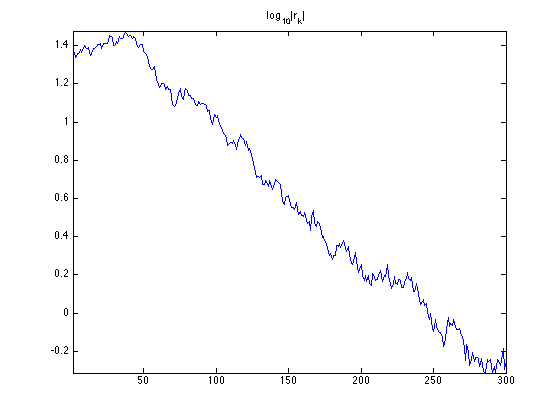## Gradient and Divergence of Images

Local differential operators like gradient, divergence and laplacian are the building blocks for variational image processing.

Load an image $$g \in \RR^N$$ of $$N=n \times n$$ pixels.

n = 256;
g = rescale( load_image('lena',n) );


Display it.

clf;
imageplot(g);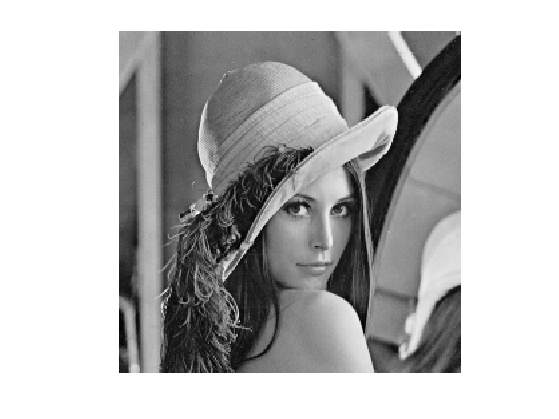For continuous functions, the gradient reads $\nabla g(x) = \pa{ \pd{g(x)}{x_1}, \pd{g(x)}{x_2} } \in \RR^2.$ (note that here, the variable $$x$$ denotes the 2-D spacial position).

We discretize this differential operator using first order finite differences. $(\nabla g)_i = ( g_{i_1,i_2}-g_{i_1-1,i_2}, g_{i_1,i_2}-g_{i_1,i_2-1} ) \in \RR^2.$ Note that for simplity we use periodic boundary conditions.

Compute its gradient, using finite differences.

s = [n 1:n-1];
grad = @(f)cat(3, f-f(s,:), f-f(:,s));


One thus has $$\nabla : \RR^N \mapsto \RR^{N \times 2}.$$

v = grad(g);


One can display each of its components.

clf;
imageplot(v(:,:,1), 'd/dx', 1,2,1);
imageplot(v(:,:,2), 'd/dy', 1,2,2);One can also display it using a color image.

clf;
imageplot(v);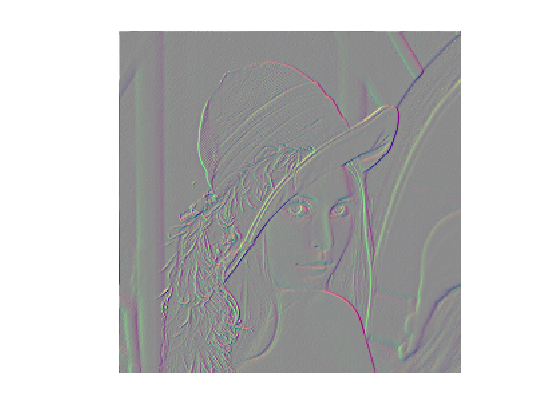One can display its magnitude $$\norm{\nabla g(x)}$$, which is large near edges.

clf;
imageplot( sqrt( sum3(v.^2,3) ) );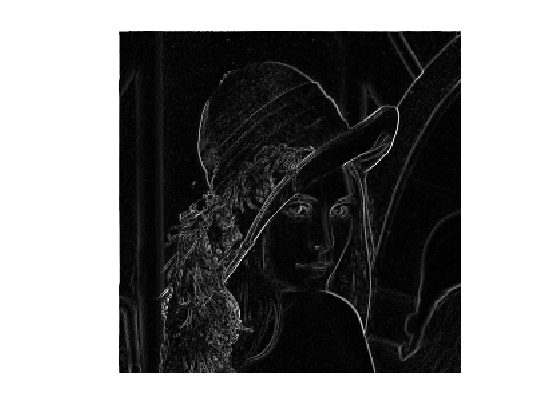The divergence operator maps vector field to images. For continuous vector fields $$v(x) \in \RR^2$$, it is defined as $\text{div}(v)(x) = \pd{v_1(x)}{x_1} + \pd{v_2(x)}{x_2} \in \RR.$ (note that here, the variable $$x$$ denotes the 2-D spacial position). It is minus the adjoint of the gadient, i.e. $$\text{div} = - \nabla^*$$.

It is discretized, for $$v=(v^1,v^2)$$ as $\text{div}(v)_i = v^1_{i_1+1,i_2} - v^1_{i_1,i_2} + v^2_{i_1,i_2+1} - v^2_{i_1,i_2} .$

t = [2:n 1];
div = @(v)v(t,:,1)-v(:,:,1) + v(:,t,2)-v(:,:,2);


The Laplacian operatore is defined as $$\Delta=\text{div} \circ \nabla = -\nabla^* \circ \nabla$$. It is thus a negative symmetric operator.

delta = @(f)div(grad(f));


Display $$\Delta f_0$$.

clf;
imageplot(delta(g));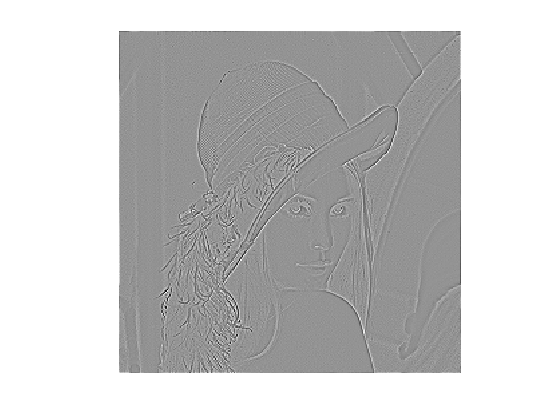Check that the relation $$\norm{\nabla f} = - \dotp{\Delta f}{f}.$$

dotp = @(a,b)sum(a(:).*b(:));
fprintf('Should be 0: %.3i\n', dotp(grad(g), grad(g)) + dotp(delta(g),g) );

Should be 0: 000


## Conjugate Gradient for Lagrangian Inpainting

We consider here the inpainting problem, which corresponds to the interpolation of missing data in the image.

We define a binary mask $$M \in \RR^N$$ where $$M_i=0$$ if the pixel indexed by $$i$$ is missing, and $$M_i=1$$ otherwise. We consider here random missing pixel, and a large missing region in the upper left corner.

M = rand(n)>.7;
w = 30;
M(end/4-w:end/4+w,end/4-w:end/4+w) = 0;


Define the degradation operator $$\Phi : \RR^N \rightarrow \RR^N$$, that corresponds to the masking with $$M$$, i.e. a diagonal operator $\Phi = \text{diag}_i(M_i).$

Phi = @(x)M.*x;


Compute the observations $$y = \Phi(x)$$ with damaged pixels.

y = Phi(g);


Display the observed image.

clf;
imageplot(y);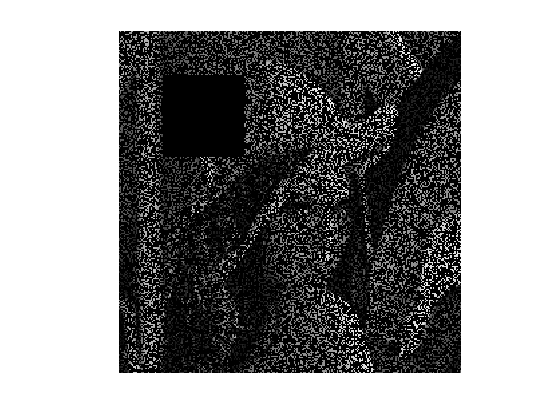To perform the recovery of an image from the damaged observations $$y$$, we aim at finding an image $$x$$ that agrees as much with the measurements, i.e. $$\Phi x \approx y$$, but at the same time is smooth. We measure the smoothness using the norm of the gradient $$\norm{\nabla x}^2$$, which corresponds to a discret Sobolev norm.

This leads us to consider the following quadratic minimization problem $\umin{x \in \RR^N} F(x) = \norm{y-\Phi x}^2 + \la \norm{\nabla x}^2.$

This problem as a unique solution if $$\text{ker}(\Phi) \cap \text{ker}(\nabla) = \{0\}$$. This condition holds in our case since $$\text{ker}(\nabla)$$ is the set of constant images.

The solution can be obtained by solving the following linear system $A x = b \qwhereq \choice{ A = \Phi^*\Phi - \la \Delta, \\ b = \Phi^* y. }$ Here we can remark that for the inpainting problem, $$\Phi^*\Phi=\Phi$$ and $$\Phi^*y=y$$.

The value of the parameter $$\lambda$$ should be small.

lambda = .01;


Operator to invert.

A = @(x)Phi(x) - lambda*delta(x);


Right hand side of the linear system is $b = \Phi^*(y) = y.$

b = y;


Exercice 2: (check the solution) Implement the conjugate gradient method, and monitor the decay of the energy $$F(x_k)$$.

exo2;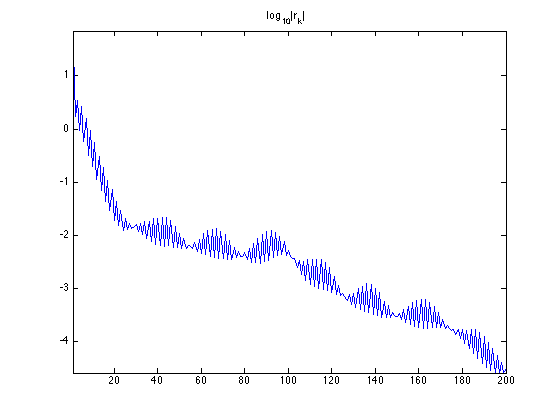Display the result.

clf;
imageplot(clamp(x));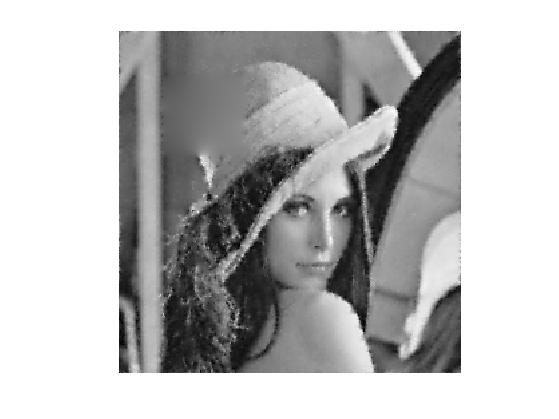## Conjugate Gradient for Constrained Inpainting

Since there is no noise perturbating the observation, it makes sense to use a $$\lambda$$ that is as small as possible.

When $$\lambda \rightarrow 0$$, the problem becomes $\umin{\Phi x = y} \norm{\nabla x}^2.$ This problem as a unique solution if $$y\in \text{Im}(\Phi)$$ and if $$\text{ker}(\Phi) \cap \text{ker}(\nabla) = \{0\}$$. This condition holds in our case, as we have already seen.

Introducing Lagrange multiplizers $$u \in \RR^N$$, this problem is equivalent to the resolution of the following couple of linear equations $\choice{ -\Delta x + \Phi^* u = 0, \\ \Phi x = y. }$

This corresponds to a single linear system over $$z = (x,u) \in \RR^{N} \times \RR^N \sim \RR^{N \times 2}$$ $A z = b \qwhereq A = \begin{pmatrix} -\Delta & \Phi^* \\ \Phi & 0 \end{pmatrix} \qandq b = \begin{pmatrix} 0 \\ y \end{pmatrix}$

Define the operator $$A$$. Note that $$x$$ is encoded in z(:,:,1) and $$u$$ in z(:,:,2).

A = @(z)cat(3, -delta(z(:,:,1)) + Phi(z(:,:,2)), Phi(z(:,:,1)) );


Define the right hand side $$b$$.

b = cat(3, zeros(n), y);


Exercice 3: (check the solution) Implement the conjugate gradient method, and monitor the decay of the energy $$F(x_k)) = \norm{\nabla x_k}$$ and the constraint $$C(x_k) = \norm{y-\Phi x_k}^2$$. Important: be carefull at the initialization of the method.

exo3;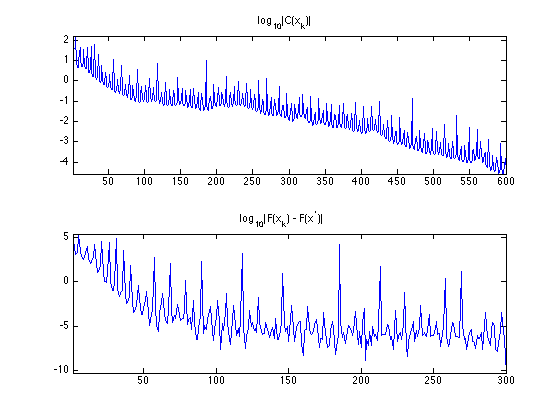Display the result.

clf;
imageplot(x);